# RD Sharma Solutions -Ex-16.4 (Part - 2), Circles, Class 9, Maths Notes | Study RD Sharma Solutions for Class 9 Mathematics - Class 9

## Class 9: RD Sharma Solutions -Ex-16.4 (Part - 2), Circles, Class 9, Maths Notes | Study RD Sharma Solutions for Class 9 Mathematics - Class 9

The document RD Sharma Solutions -Ex-16.4 (Part - 2), Circles, Class 9, Maths Notes | Study RD Sharma Solutions for Class 9 Mathematics - Class 9 is a part of the Class 9 Course RD Sharma Solutions for Class 9 Mathematics.
All you need of Class 9 at this link: Class 9

Q5) O is the circumference of the triangle ABC and Odis perpendicular on BC. Prove that  ∠BOD = ∠A.

Solution:

Given O is the circum centre of triangle ABC and OD⊥BC

to prove  ∠BOD = 2 ∠A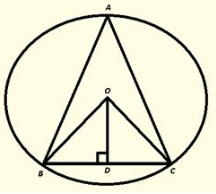Pro of :

In ΔOBD and ΔOCD
∠ODB = ∠ODC [Each900]
OB = OC [Radius of circle]
OD = OD [Common]

ThenΔOBD≅ΔOCD         [ by RHS Condition] .

∴ ∠BOD = ∠COD…..(i)
[PCT] .  by degree measure theorem ∠BOC = 2 ∠BAC
⇒ 2 ∠BOD = 2 ∠BAC [ by using(i)]
⇒ ∠BOD = ∠BAC.

Q6) In figure 16.135, O is the centre of the circle, BO is the bisector of   ∠ABC. Show that AB = AC.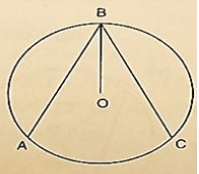Solution:

Given, BO is the bisec to r of   ∠ABC

to prove AB = BC

Pro of :

Since, BO is the bisec to r of   ∠ABC.

Then,  ∠ABO = ∠CBO…..(i)

Since, OB = OA                     [Radius of circle]

Then,  ∠ABO = ∠DAB…..(ii)             [opposite Angles to equal sides]

Since OB = OC                       [Radius of circle]

Then,  ∠OAB = ∠OCB…..(iii)  [opposite Angles to equal sides]

Compare equations (i), (ii) and (iii)

∠OAB = ∠OCB…..(iv)

InΔOAB andΔOCB

∠OAB = ∠OCB                      [From (iv)]

∠OBA = ∠OBC                      [Given]

OB = OB                                                                    [Common]

Then, ΔOAB≅ΔOCB [ by AAScondition]
∴AB = BC [CPCT]

Q7) In figure 16.136, O is the centre of the circle, then prove that  ∠x = ∠y+ ∠z.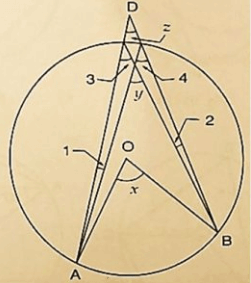Solution:

We have,

∠3 = ∠4 [ Angles insamesegment]
∴ ∠x = 2 ∠3 [ by degree measure theorem]
⇒ ∠x = ∠3+ ∠3
⇒ ∠x = ∠3+ ∠4…..(i)             [ ∠3 = angle4]
But ∠y = ∠3+ ∠1 [ by exteriorangleproperty]
⇒ ∠3 = ∠y− ∠1…..(ii)
from(i) and(ii)
∠x = ∠y− ∠1+ ∠4
⇒ ∠x = ∠y+ ∠4− ∠1
⇒ ∠x = ∠y+ ∠z+ ∠1− ∠1 [ by exteriorangleproperty]
⇒ ∠x = ∠y+ ∠z

Q8) In figure 16.137, O and O’ are centers of two circles intersecting at B and C. ACD is a straight line, find x.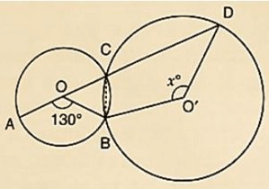Solution:

by degree measure theorem ∠AOB = 2 ∠ACB
⇒ 1300 = 2 ∠ACB
⇒ ∠ACB =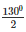= 650
∴ ∠ACB+ ∠BCD = 1800 [Linerapair of Angles ]
⇒ 650+ ∠BCD = 1800
⇒ ∠BCD = 1800−65= 1150 by degree measure theoremreflex ∠BOD = 2 ∠BCD
⇒ reflex ∠BOD = 2×1150 = 2300
Now,reflex ∠BOD+ ∠BO′D = 3600 [Complexangle]
⇒ 2300+x = 3600
⇒ x = 3600−2300
∴x = 1300

Q9) In figure 16.138, O is the centre of a circle and PQ is a diameter. If  ∠ROS = 400,find ∠RTS..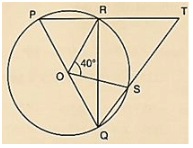Solution:

Since PQ is diameter

Then,

∠PRQ = 900 [Angleinsemicircle]
∴ ∠PRQ+ ∠TRQ = 1800 [Linearpair of angle]
900+ ∠TRQ = 1800
∠TRQ = 1800−900 = 900.

by degree measure theorem

∠ROS = 2 ∠RQS

⇒ 400 = 2 ∠RQS

⇒ ∠RQS =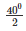= 200

In ΔRQT, by Angle sum property

∠RQT+ ∠QRT+ ∠RTS = 1800

⇒ 200+900+ ∠RTS = 1800

⇒ ∠RTS = 1800−200−90= 700

Q10) In figure 16.139, if  ∠ACB = 400, ∠DPB = 1200,find ∠CBD.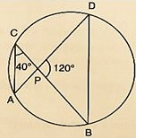Solution:

We have,

∠ACB = 400; ∠DPB = 1200

∴ ∠APB = ∠DCB = 400 [Angleinsamesegment]
InΔPOB, by Angles umproperty ∠PDB+ ∠PBD+ ∠BPD = 1800
⇒ 400+ ∠PBD+1200 = 1800
⇒ ∠PBD = 1800−400−1200
⇒ ∠PBD = 200
∴ ∠CBD = 200

Q11) A chord of a circle is equal to the radius of the circle. Find the angle subtended by the chord at a point on the minor arc and also at a point on the major arc.

Solution:

We have,

= >OA = OB = AB

Then triangle OAB is an equilateral triangle.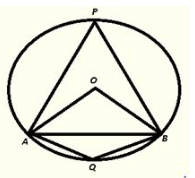∴ ∠AOB = 600 [oneangle of equilateraltriangle]

by degree measure theorem

∠AOB = 2 ∠APB

⇒ 600 = 2 ∠APB

⇒ ∠APB =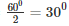Now, ∠APB+ ∠AQB = 1800 [opposite Angles of cyclicquadrilateral]

⇒ 300+ ∠AQB = 1800

⇒ ∠AQB = 1800−300 = 1500.

Therefore, Angle by chord AB at minor arc = 1500

Angle by chord AB at major arc = 300

The document RD Sharma Solutions -Ex-16.4 (Part - 2), Circles, Class 9, Maths Notes | Study RD Sharma Solutions for Class 9 Mathematics - Class 9 is a part of the Class 9 Course RD Sharma Solutions for Class 9 Mathematics.
All you need of Class 9 at this link: Class 9Use Code STAYHOME200 and get INR 200 additional OFF

## RD Sharma Solutions for Class 9 Mathematics

91 docs

Track your progress, build streaks, highlight & save important lessons and more!

,

,

,

,

,

,

,

,

,

,

,

,

,

,

,

,

,

,

,

,

,

,

,

,

,

,

,

,

,

,

;